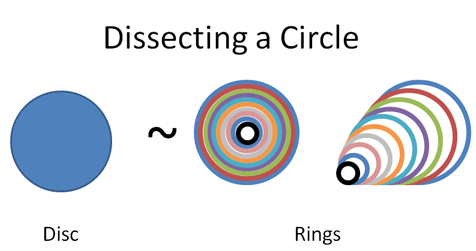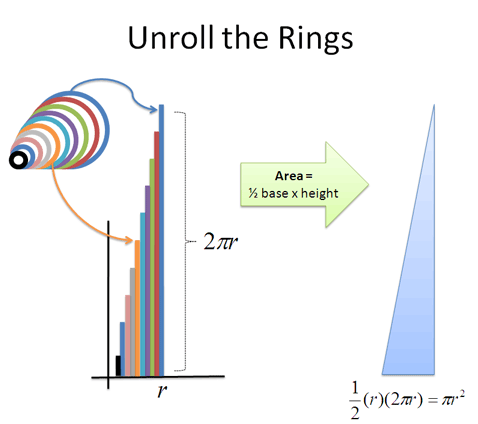# Calculus Learning Guide

This is a realistic learning plan for Calculus based on the ADEPT method.

# I have a few minutes for Calculus, what can I learn?

## 1 minute: The Big Aha!

Level 1: Appreciation

Calculus is the art of splitting patterns apart (X-rays, derivatives) and gluing patterns together (Time-lapses, integrals). Sometimes we can cleverly re-arrange the pattern to find a new insight.

A circle can be split into rings:And the rings turned into a triangle:Wow! We found the circle's area in a simpler way. Welcome to Calculus.

Checkpoint:

• Do you want to learn more more?

## +20 minutes: Intuitive Appreciation

Level 2: Natural Description

Checkpoint: Describe, in your own words:

• What Calculus does
• X-Ray Vision
• Time-lapse Vision
• The tradeoffs when splitting a circle into rings, wedges, or boards
• How to build a 3d shape from 2d parts

## +20 minutes: Technical Description

Level 3: Symbolic Description

Checkpoint: Describe, in your own words:

• Integral
• Derivative
• Integrand (a single step)
• Bounds of integration

Skills:

• Describe a Calculus action (splitting a circle into rings) using the official language
• Enter the official language into Wolfram Alpha to solve the problem

## +30 minutes: Theory I

Level 4: Basic Theory

Checkpoint: Describe, in your own words:

• How integrals/derivatives relate to multiplication/division

Skills:

• Find the derivative/integral of a line
• Find the derivative/integral of a constant
• Find the derivative/integral of a square
• Recognize the common notations for the derivative
• Estimate the change in f(x) = x2 using a step of size dx

## +1 hour: Theory II

Checkpoint: Describe, in your own words:

• How an infinite process can have a finite result
• How a process with limited precision can point to a perfect result
• The formal definition of the derivative
• Estimate the change in f(x) = x2 using a step of size dx, and let dx go to zero. Verify the limit using Wolfram Alpha.
• The Fundamental Theorem of Calculus (FTOC)

Derive and put into your own words:

• The addition rule: (f + g)' = ?
• The product rule: (f · g)' = ?
• The inverse rule: (frac(1)(x))' = ?
• The power rule: (xn)' = ?
• The quotient rule: (frac(f)(g))' = ?
• Solve frac(d)(dx) 3x5 on your own and verify with Wolfram Alpha
• Solve int 2x2 on your own and verify with Wolfram Alpha

## +1 hour: Basic Problem Solving

Level 5: Basic Performance

Checkpoint: Describe how to turn the circumference of a circle into the area of a circle:

• Explain your plan in plain English
• Explain your plan using the official math notation
• Apply the rules of Calculus to your equation and calculate the result
• Verify the result using Wolfram Alpha
• Repeat the steps above, turning the area of a circle into the volume of a sphere
• Repeat the steps above, turning the volume of a sphere into the surface area of a sphere

Read the lessons above in Calculus, Better Explained, now an Amazon bestseller.

## +12 weeks: I need to pass a course!

Level 5: Advanced Performance

Gotcha. The best use of time is still spending a few hours on the above goals, to build a solid intuition. Then, begin your Calculus course, such as:

• Elementary Calculus: An Infinitesimal Approach by Jerome Keisler (2002). This book is based on infinitesimals (an alternative to limits, which I like) and has plenty of practice problems. Available in print or free online.

• Calculus Made Easy by Silvanus Thompson (1914). This book follows the traditional limit approach, and is written in a down-to-earth style. Available on Project Gutenberg and print.

• MIT 1801: Single Variable Calculus. Includes video lectures, assignments, exams, and solutions. Available free online.

As you go through the traditional course, keep this in mind:

• Review the intuitive definition. Rephrase technical definitions in terms that make sense to you.

• It's completely fine to use online tools for help. When stuck, get a hint, fix your mistakes, and try solving a new problem on your own.

• Relate graphs back to shapes. Most courses emphasize graphs and slopes; convert the concepts to shapes to help visualize them.

• Skip limits if you get stuck. Limits (and infinitesimals) were invented after the majority of Calculus. If you struggle, move on and return later.